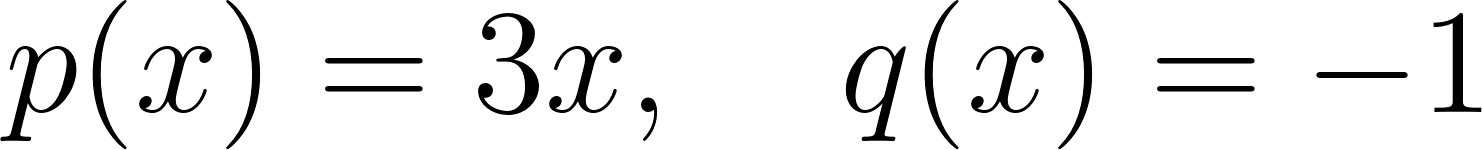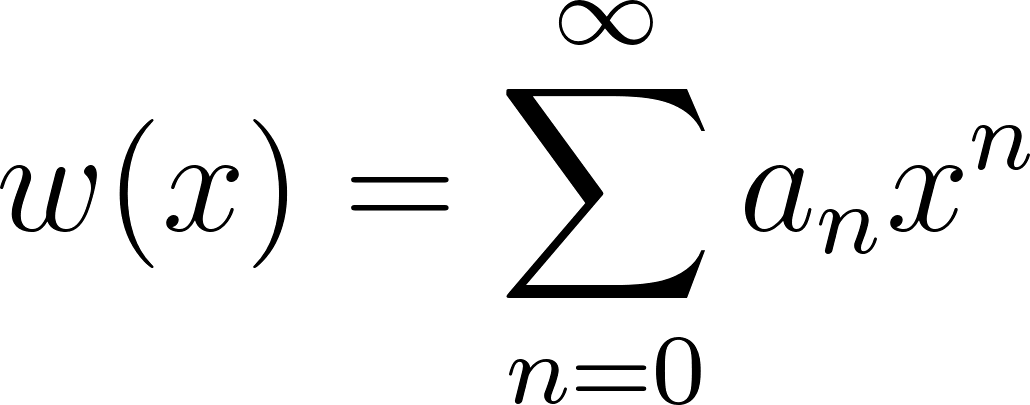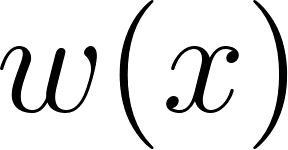×
Get Full Access to Fundamentals Of Differential Equations - 8 Edition - Chapter 8.3 - Problem 25e
Get Full Access to Fundamentals Of Differential Equations - 8 Edition - Chapter 8.3 - Problem 25e

×

# In 25–28, find at least the first four nonzero terms in aISBN: 9780321747730 43

## Solution for problem 25E Chapter 8.3

Fundamentals of Differential Equations | 8th Edition

• Textbook Solutions
• 2901 Step-by-step solutions solved by professors and subject experts
• Get 24/7 help from StudySoup virtual teaching assistantsFundamentals of Differential Equations | 8th Edition

4 5 1 414 Reviews
15
3
Problem 25E

In Problems 25–28, find at least the first four nonzero terms in a power series expansion about $$x=0$$ for the solution to the given initial value problem.

$$w^{\prime \prime}+3 x w^{\prime}-w=0$$

$$w(0)=2, w^{\prime}(0)=0$$

Equation Transcription:Text Transcription:

x=0

w''+3xw'-w=0

w(0)=2, w'(0)=0

Step-by-Step Solution:

Step 1 of 6

We know thatTherefore, it has no singular points which means thatis an ordinary point.

Consider a solution of the form:To substitutein the given equation, we need to find its first and second derivative, with respect to.

Step 2 of 6

Step 3 of 6

##### ISBN: 9780321747730

This textbook survival guide was created for the textbook: Fundamentals of Differential Equations , edition: 8. The full step-by-step solution to problem: 25E from chapter: 8.3 was answered by , our top Calculus solution expert on 07/11/17, 04:37AM. Fundamentals of Differential Equations was written by and is associated to the ISBN: 9780321747730. Since the solution to 25E from 8.3 chapter was answered, more than 382 students have viewed the full step-by-step answer. The answer to “?In 25–28, find at least the first four nonzero terms in a power series expansion about $$x=0$$ for the solution to the given initial value problem.$$w^{\prime \prime}+3 x w^{\prime}-w=0$$$$w(0)=2, w^{\prime}(0)=0$$Equation Transcription:Text Transcription:x=0w''+3xw'-w=0w(0)=2, w'(0)=0” is broken down into a number of easy to follow steps, and 33 words. This full solution covers the following key subjects: Expansion, Find, given, initial, least. This expansive textbook survival guide covers 67 chapters, and 2118 solutions.

Unlock Textbook Solution